# Sampling Theorem Antialiasing Motivations My ray traced images

• Slides: 35Sampling Theorem & Antialiasing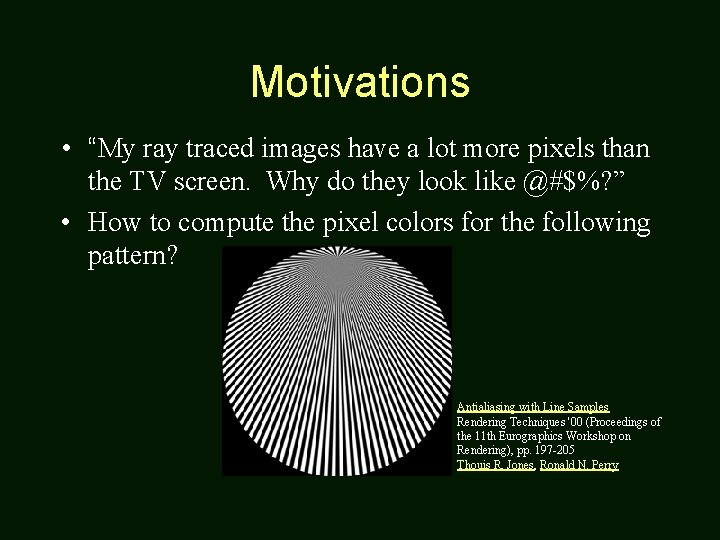Motivations • “My ray traced images have a lot more pixels than the TV screen. Why do they look like @#\$%? ” • How to compute the pixel colors for the following pattern? Antialiasing with Line Samples Rendering Techniques '00 (Proceedings of the 11 th Eurographics Workshop on Rendering), pp. 197 -205 Thouis R. Jones, Ronald N. Perry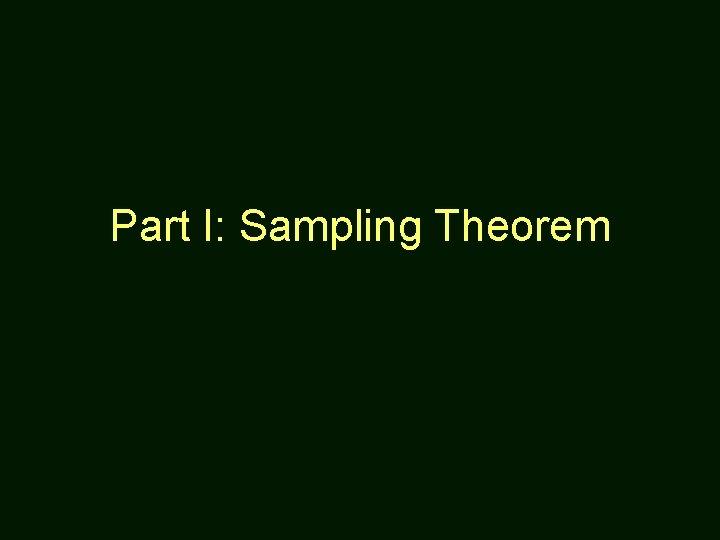Part I: Sampling TheoremExample of Aliasing in Computer GraphicsExamples of Aliasing in 1 D • See Figure 14. 2 (p. 394) of Watt’s book for other examples.An Intuition – Using a Single Frequency • It’s easy to figure out for a sin wave. • What about any signal (usually a mixture of multiple frequencies)? • Enter Fourier Transform…Sampling • 1 D Signal: x f(x) becomes i f(i) • 2 D Image: x, y f(x, y) – For grayscale image, f(x, y) is the intensity of pixel at (x, y).Reconstruction • If the samples are “dense” enough, then we can recover the original signal. • Question is: How dense is enough?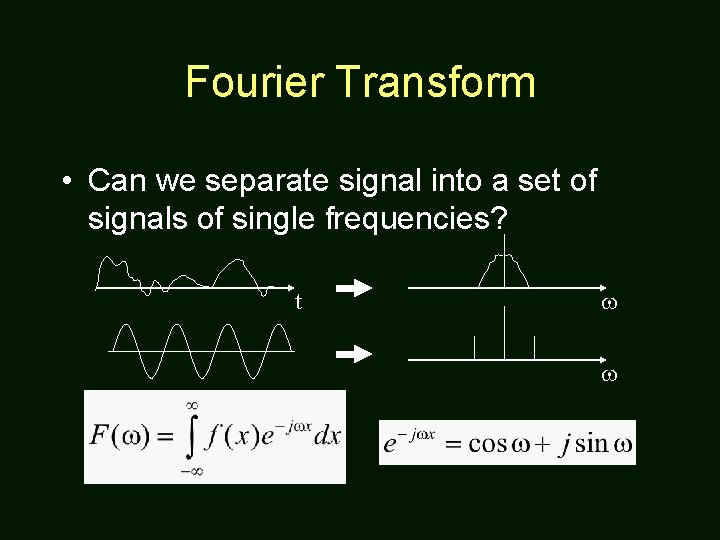Fourier Transform • Can we separate signal into a set of signals of single frequencies? t w w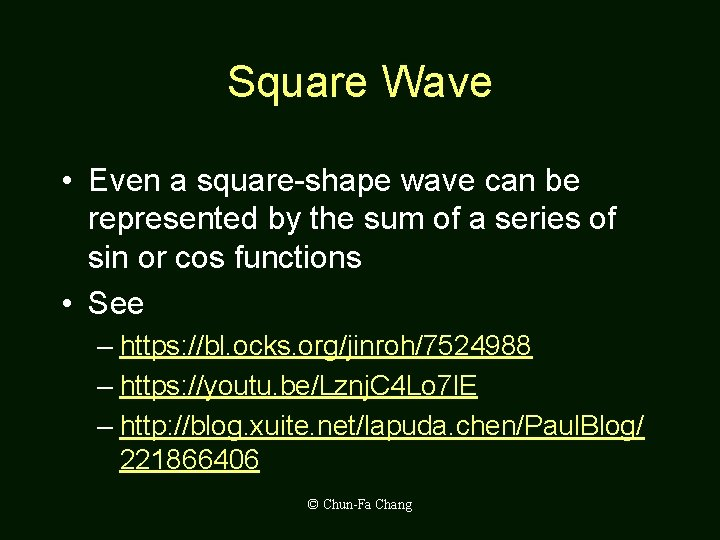Square Wave • Even a square-shape wave can be represented by the sum of a series of sin or cos functions • See – https: //bl. ocks. org/jinroh/7524988 – https: //youtu. be/Lznj. C 4 Lo 7 l. E – http: //blog. xuite. net/lapuda. chen/Paul. Blog/ 221866406 © Chun-Fa ChangBasis Functions • An example: X=[x 1, x 2, …, xn] U=[u 1, u 2, …, un] V=[v 1, v 2, …, vn] Let X = a*U + b*V, how to find a and b? • If U and V are orthogonal, then a and b are the projection of X onto U and V.Compared to Fourier Transform • Consider a continuous signal as a infinitedimensional vector [ f(e), f(2 e), f(3 e), …. . ] t • Consider each frequency w a basis, then F(w) is the projection of f(x) onto that basis.Sampling • Spatial domain: multiply with a pulse train. • Frequency domain: convolution!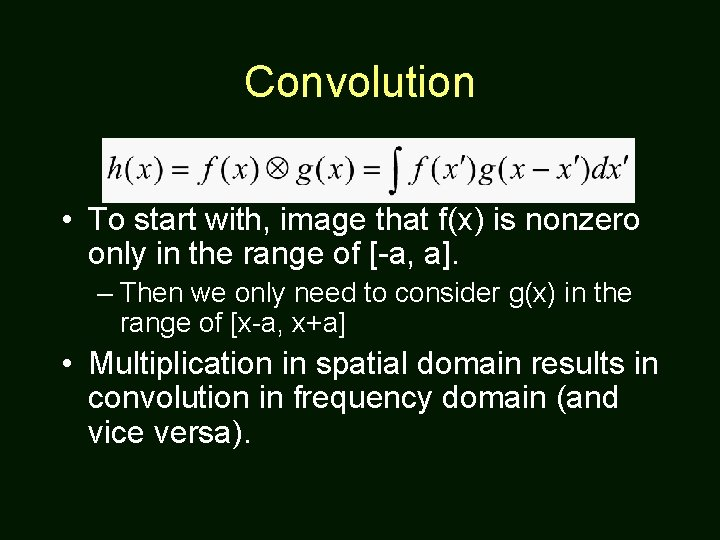Convolution • To start with, image that f(x) is nonzero only in the range of [-a, a]. – Then we only need to consider g(x) in the range of [x-a, x+a] • Multiplication in spatial domain results in convolution in frequency domain (and vice versa).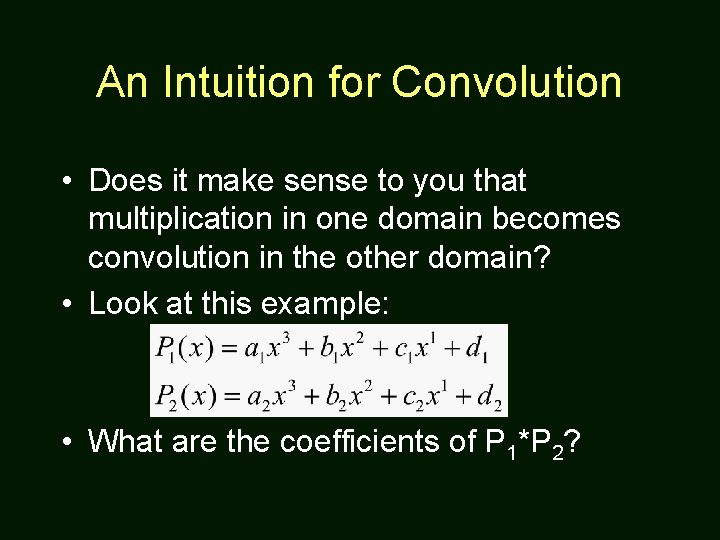An Intuition for Convolution • Does it make sense to you that multiplication in one domain becomes convolution in the other domain? • Look at this example: • What are the coefficients of P 1*P 2?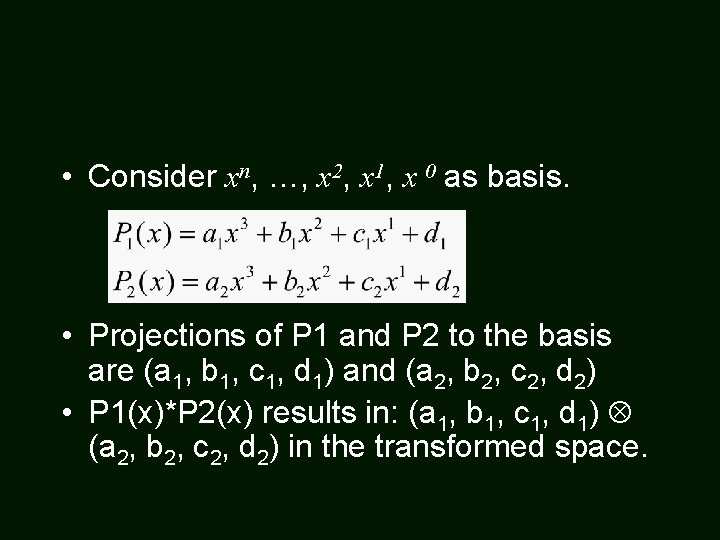• Consider xn, …, x 2, x 1, x 0 as basis. • Projections of P 1 and P 2 to the basis are (a 1, b 1, c 1, d 1) and (a 2, b 2, c 2, d 2) • P 1(x)*P 2(x) results in: (a 1, b 1, c 1, d 1) (a 2, b 2, c 2, d 2) in the transformed space.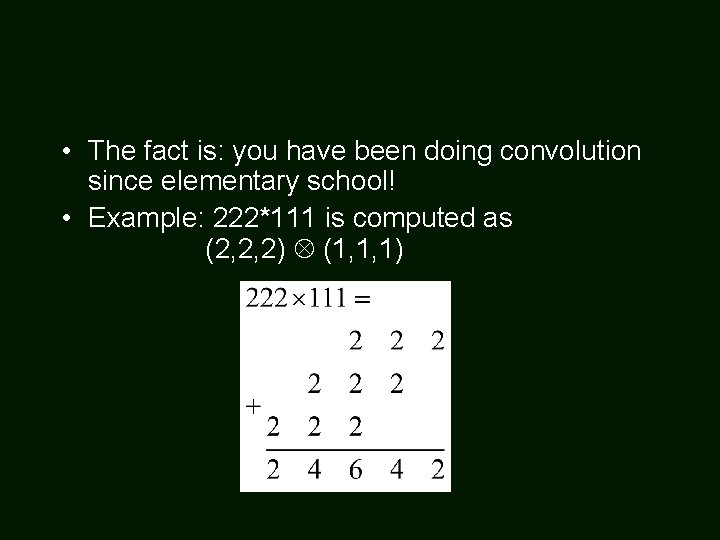• The fact is: you have been doing convolution since elementary school! • Example: 222*111 is computed as (2, 2, 2) (1, 1, 1)Reconstruction • Frequency domain: • Spatial domain: convolve with Sinc function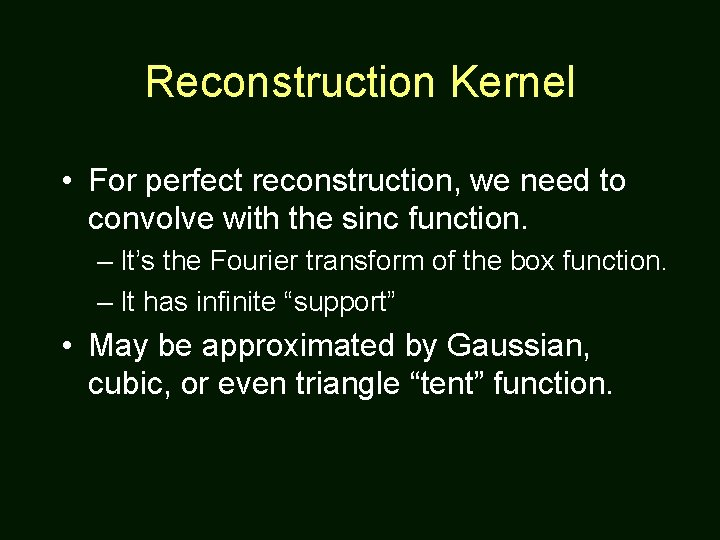Reconstruction Kernel • For perfect reconstruction, we need to convolve with the sinc function. – It’s the Fourier transform of the box function. – It has infinite “support” • May be approximated by Gaussian, cubic, or even triangle “tent” function.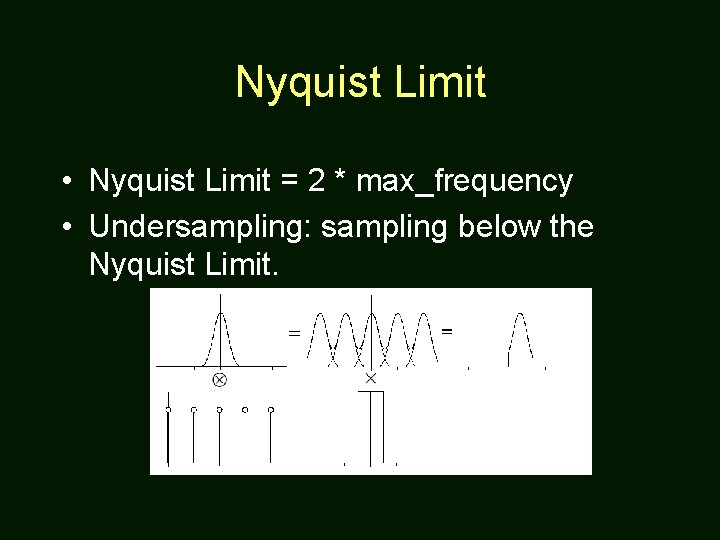Nyquist Limit • Nyquist Limit = 2 * max_frequency • Undersampling: sampling below the Nyquist Limit.Part II: AntialiasingChanges within a Pixel • A lot can change within a pixel: – Shading – Edge – Texture • Point sampling at the center often produces undesirable result.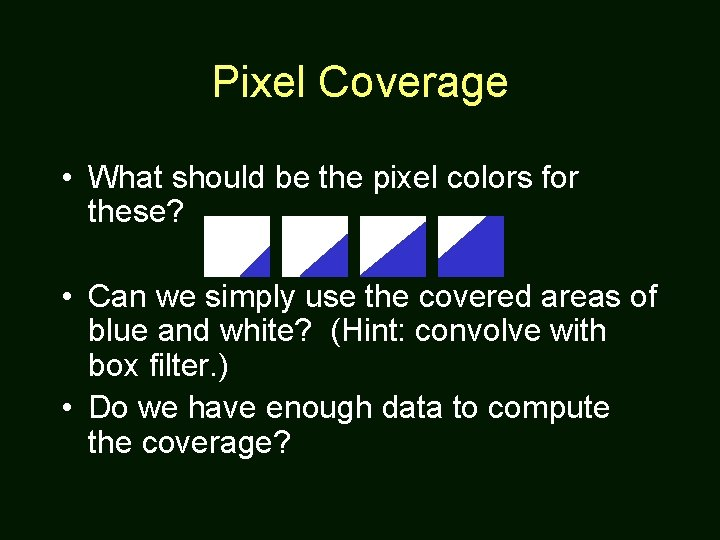Pixel Coverage • What should be the pixel colors for these? • Can we simply use the covered areas of blue and white? (Hint: convolve with box filter. ) • Do we have enough data to compute the coverage?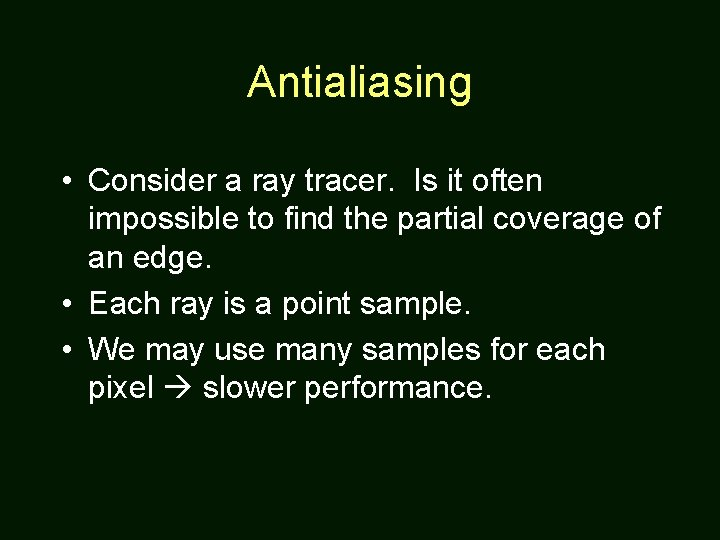Antialiasing • Consider a ray tracer. Is it often impossible to find the partial coverage of an edge. • Each ray is a point sample. • We may use many samples for each pixel slower performance.Antialiasing – Uniform Sampling • Also called supersampling • Wasteful if not much changes within a pixel.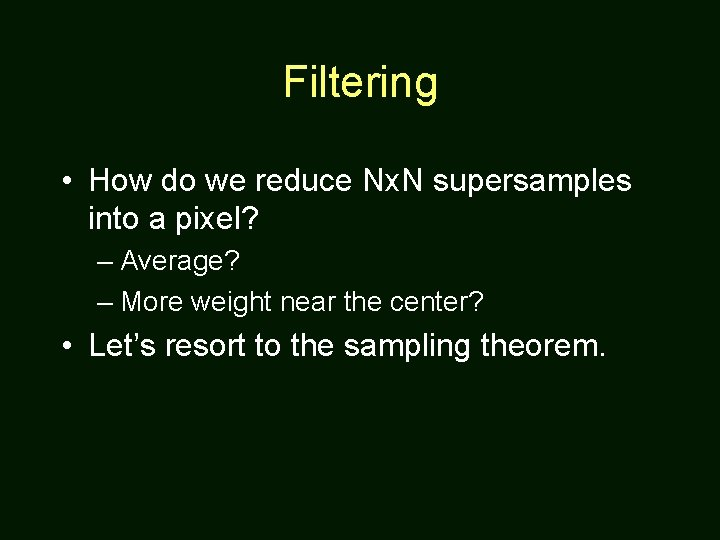Filtering • How do we reduce Nx. N supersamples into a pixel? – Average? – More weight near the center? • Let’s resort to the sampling theorem.Reconstruction • Frequency domain: • Spatial domain: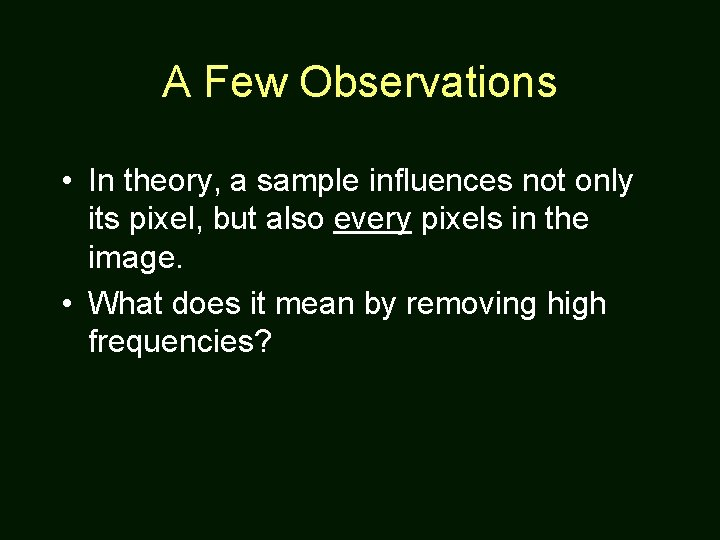A Few Observations • In theory, a sample influences not only its pixel, but also every pixels in the image. • What does it mean by removing high frequencies?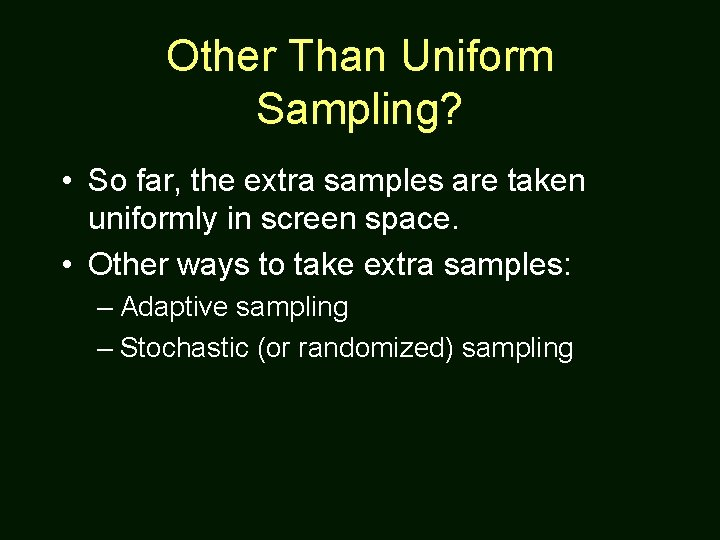Other Than Uniform Sampling? • So far, the extra samples are taken uniformly in screen space. • Other ways to take extra samples: – Adaptive sampling – Stochastic (or randomized) samplingAntialiasing – Adaptive Sampling • Feasible in software, but difficult to implement in hardware. • Increase samples only if necessary. • But how do we know when is “necessary”? – Check the neighbors.Antialiasing – Stochastic Sampling • Keep the same number of samples per pixel. • Replace the aliasing effects with noise that is easier to ignore.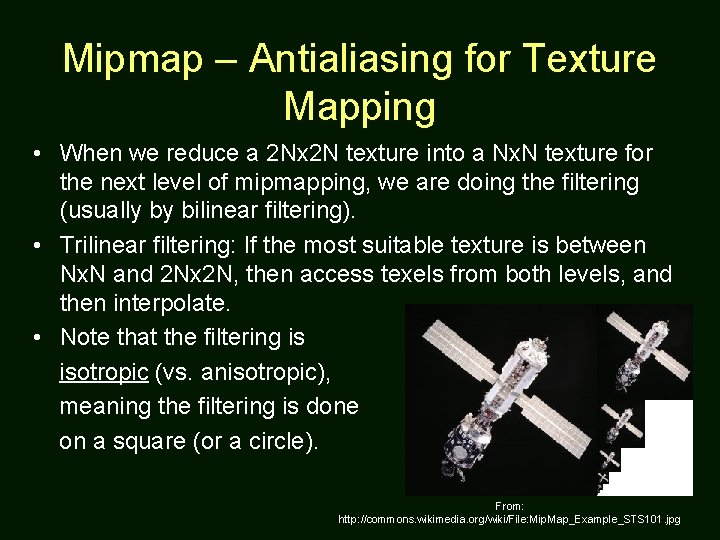Mipmap – Antialiasing for Texture Mapping • When we reduce a 2 Nx 2 N texture into a Nx. N texture for the next level of mipmapping, we are doing the filtering (usually by bilinear filtering). • Trilinear filtering: If the most suitable texture is between Nx. N and 2 Nx 2 N, then access texels from both levels, and then interpolate. • Note that the filtering is isotropic (vs. anisotropic), meaning the filtering is done on a square (or a circle). From: http: //commons. wikimedia. org/wiki/File: Mip. Map_Example_STS 101. jpg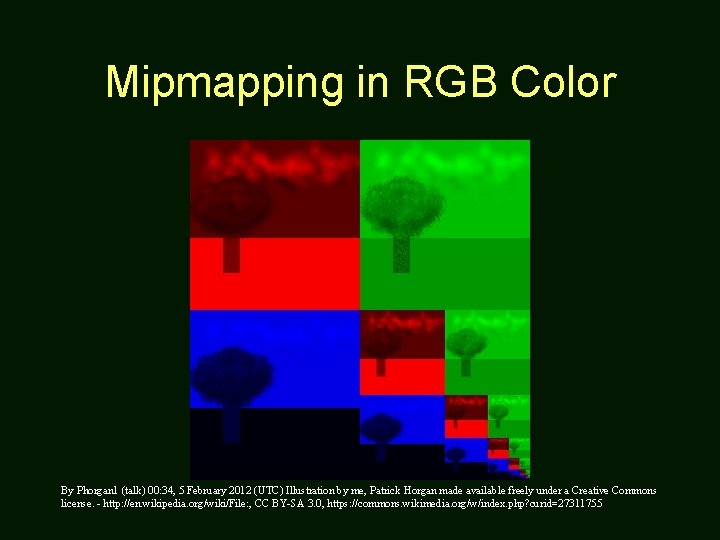Mipmapping in RGB Color By Phorgan 1 (talk) 00: 34, 5 February 2012 (UTC) Illustration by me, Patrick Horgan made available freely under a Creative Commons license. - http: //en. wikipedia. org/wiki/File: , CC BY-SA 3. 0, https: //commons. wikimedia. org/w/index. php? curid=27311755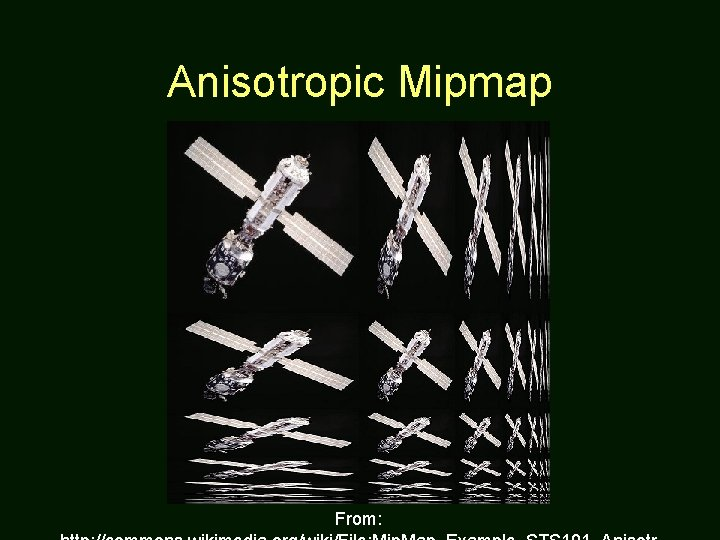Anisotropic Mipmap From: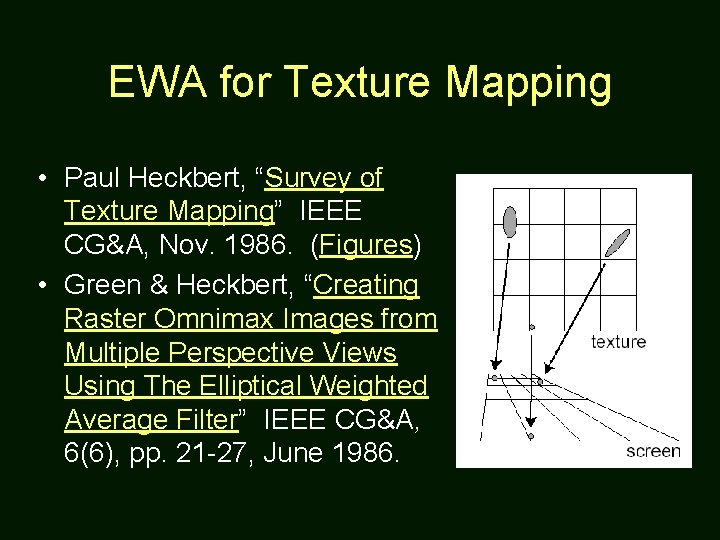EWA for Texture Mapping • Paul Heckbert, “Survey of Texture Mapping” IEEE CG&A, Nov. 1986. (Figures) • Green & Heckbert, “Creating Raster Omnimax Images from Multiple Perspective Views Using The Elliptical Weighted Average Filter” IEEE CG&A, 6(6), pp. 21 -27, June 1986.